# Subtracting with regrouping (using base ten blocks)

### Subtracting with regrouping (using base ten blocks)

In this lesson, we will learn:

• How to regroup base ten block models ("borrowing" or "exchanging")
• How to subtract using base ten block models

Notes:

• Like addition, we will also need to regroup our base ten blocks for subtraction

• When we don't have enough to subtract, we need to borrow from the next place value up to make more (splitting up the base ten block models)
• Sometimes, this is called "exchanging"

1 ten can be exchanged into 10 ones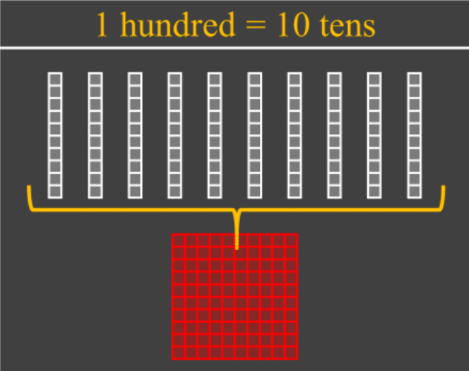1 hundred can be exchanged into 10 tens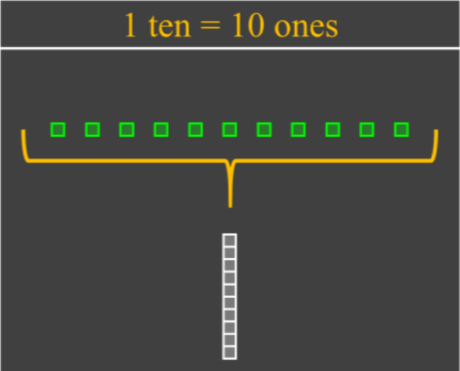1 thousand can be exchanged into 10 hundreds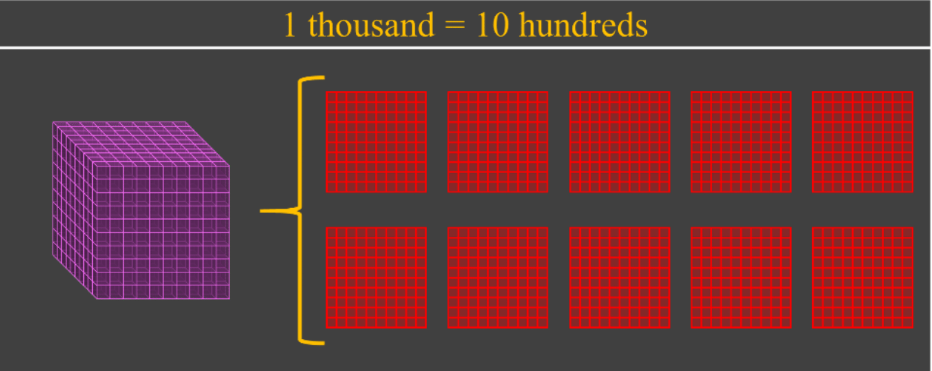#### Lessons

• Introduction
Introduction to Subtracting with Regrouping (using base ten block models):
a)
How to use base ten blocks to model numbers

b)
How to show regrouping using block models for subtraction

• 1.
Subtracting 2-digit numbers without regrouping (using base ten block models)
Subtract:
a)
16 - 4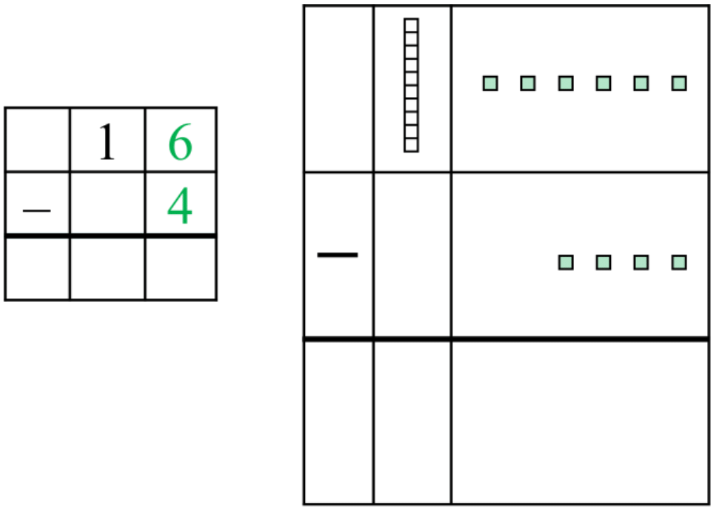b)
38 - 15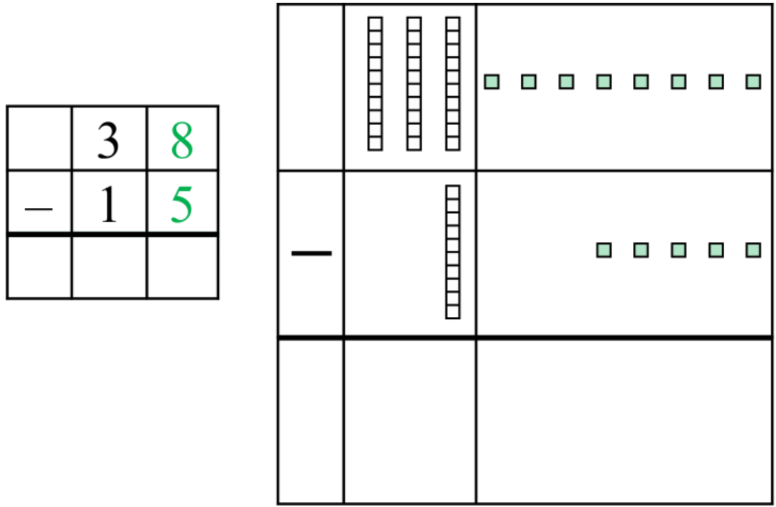• 2.
Subtracting 2-digit numbers with regrouping (using base ten blocks)
Subtract:
a)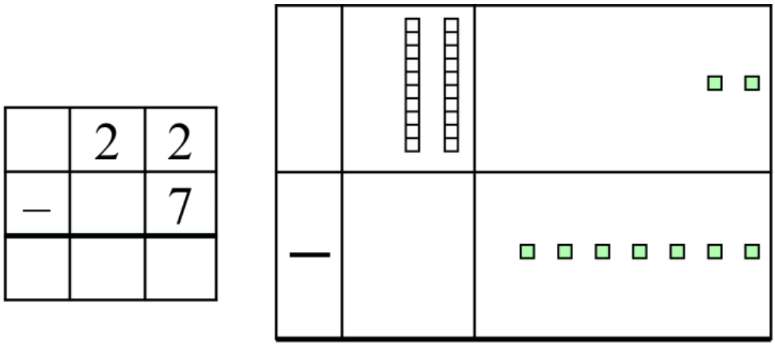b)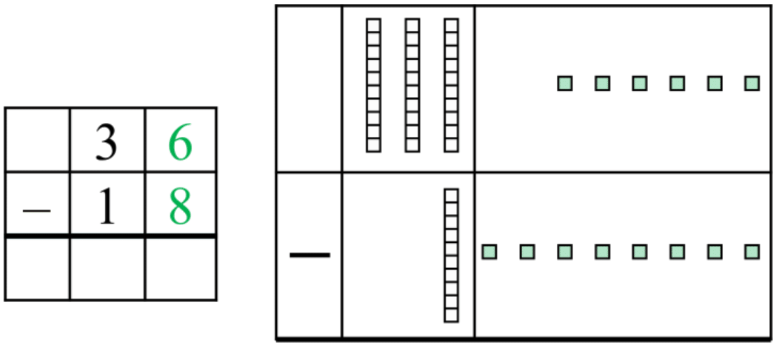• 3.
Subtracting 3-digit numbers with regrouping (using base ten blocks)
Subtract: 468 - 281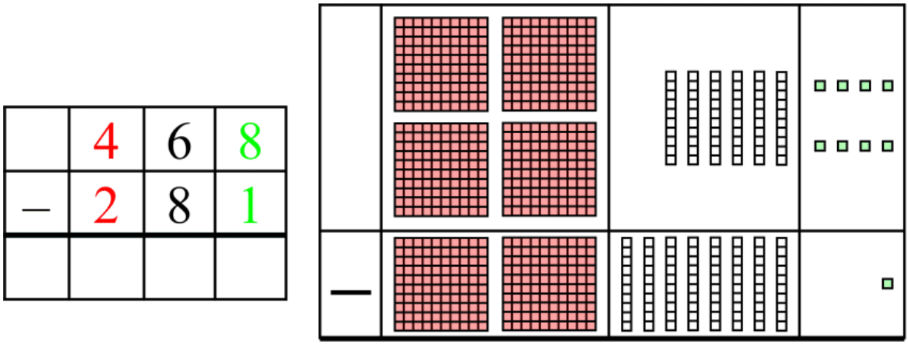• 4.
Word problem for subtracting with regrouping (with base ten block models)
On Halloween, Freddy had 159 candies to give out. After the first two hours, he had already given out 73 candies. In the next two hours, he gave out 61 more candies. How many candies does Freddy have left at the end of the night?

Use base ten block models to help you answer the question.